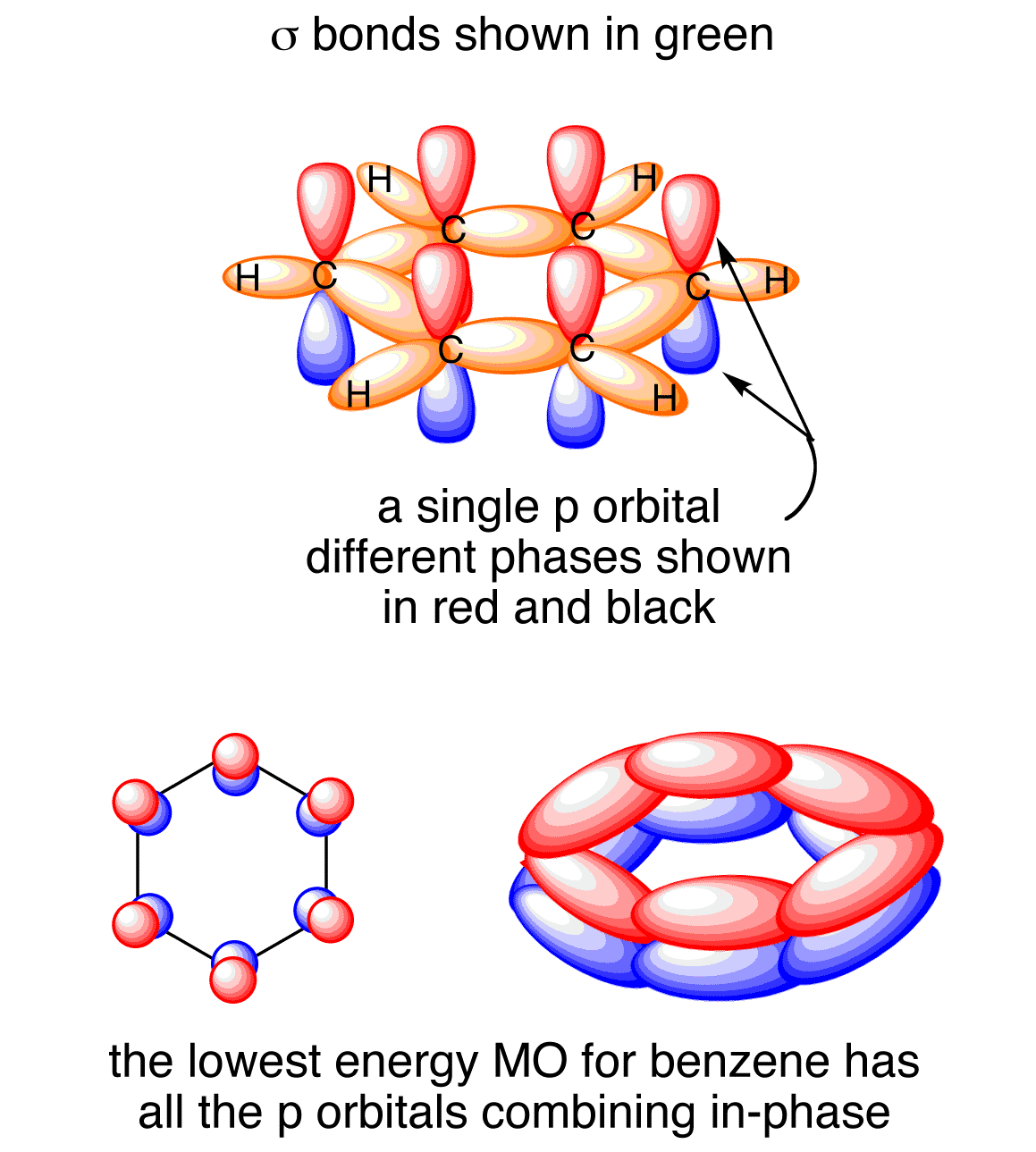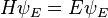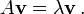# Homework at Berkeley

By other names.

You know, cuz nobody calls it HW anymore. Instead it’s “studying”, “problem sets”, “papers”, etc… just not “homework”.

Just an observation.

# An Intuitive Approach to Gauss’ Law

…One that does not use the traditional route of formally introducing electric flux first.

Recall that the density of an object is defined as the mass per unit volume. You can stretch or bend or readjust the orientation of the object all you want. Its density might change, but the mass contained within the volume of the object is conserved; no mass is created nor destroyed. And a similar density concept and conservation law will be used to derive Gauss’ Law.

We start by imagining the electric field as a very large body that is cut up into many infinitely thin [simply-connected] shells (that do not fold back on themselves or do weird things like that). Like mass, electric field lines emanating from a source (let’s say at the center of the large body) cannot be destroyed, nor cannot they spontaneously appear out of thin air. So for each thin shell, the amount of field lines does not change. We call that amount N. Intuitively, one can think of stretching a spherical surface containing N back and forth. The density of field lines (which we will define very soon) will change but the amount of field lines cutting through the surface remains the same. Just like the earlier mass example.

We will cut an arbitrary shell into many many small rectangles of area dA that “contain” a small differential amount of field lines dN. Define the field line density for the small rectangle as:

In analogue to the mass density we recalled earlier.

Multiplying both sides by dA and integrating across the shell/surface yields:

Acknowledging that any arbitrary closed shell will contain N field lines.

We’re almost done now! We just have to recall two fundamental concepts in electrostatics:
a) the amount of electric field lines – N – is proportional to the strength of the sourceQ; and
b) the closeness (“density”) of field lines – – is proportional to the strength of the field itselfE.

Making appropriate substitutions,

Or

The constant prefactor on the RHS can be experimentally determined, and it happens to be . So finally we get

Or Gauss’ Law.

QED.

(Inspired by Professor Bloxham‘s first Physics 7C lecture, which reviewed E&M.)

# Chem 3B and Physics 7B

…Combined!

Benzene is a beautiful molecule. It really is. In terms of bonding structure, it’s especially beautiful – it’s aromatic. Each carbon in the benzene molecule is sp2 bonded to another carbon and a hydrogen (or a heteroatom, if one so pleases, but we will discuss only hydrogen for reasons to be seen later). This forms the sigma bond network in the benzene ring. One remaining p orbital remains open, and it turns out that all the remaining p orbitals bond form a separate pi bond network. The remaining pi electrons are delocalized through this pi molecular orbital that circulates around the benzene ring. What I just said is illustrated in the following diagram:Not only does this delocalization afford special stability, it means any and all pi electrons can circulate through the pi molecular orbital. They can move about back and forth – kinda like electrons in a closed circuit. Now it gets interesting. Physics 7B time!

Suppose you apply an external magnetic field to this closed aromatic circuit. Because this ring is closed, there’s a non-negligible magnetic flux. As the external B field increases, the flux increases, which induces a voltage (potential difference) across the aromatic ring, producing a current across the ring. The direction of the current can be given by Lenz’s Law, which states that the induced current will produce an induced magnetic flux opposing the source flux. For instance, if the external B field points up, the induced current will flow CW to produce a magnetic field that points down in the ring.

Why does any of this matter? Because NMR spectroscopy works by introducing an external magnetic field across a molecule! In the presence of an applied magnetic field and electromagnetic radiation, nuclei will resonate at a certain frequency. This frequency is determined by the exposure of the nuclei to the magnetic field. When electron donating functional groups are located near the nuclei, the extra electron density “shields” the nuclei from the field; with electron withdrawing groups the nuclei are deshielded and exposed to the field. These are quantified in chemical shifts – the larger the shift, the greater exposure to the magnetic field.

In benzene however, the mechanism works differently. By the right hand rule, an induced B field that opposes the external field will come around and point in the direction of the external field outside the ring, where the hydrogens lie. Since we talk about 1H NMR in organic chemistry, this means that the induced current increases the hydrogen nuclei’s exposure to magnetic fields – entailing a larger chemical shift! And that is why benzene has that weird 6.0-8.5ppm shift, greater than alkenes and almost comparable to aldehyde proton shifts.

This blew my mind when I first read it (never was taught this in my organic chemistry class)… so I thought I’d share for the 0.1 readers who visit my blog. 😀

# Probability, and some Quantum Mechanics

Read God’s Debris, by Scott Adams (thanks to Charles L. for the recommendation). It’s a good read. I don’t agree with what it says because I do not believe in the same Theism but it offers up some interesting ideas. What interested me the most was its emphasis on probability.

Specifically, Adams claims that the world is governed by it. Now, this may be what we perceive the world as (a series of events each with a certain probability), but the root cause is not probability. Rather, it’s energetics. For instance, the equilibrium ratio of a state B to a state A of any reversible transformation can be tied back to how energetically favorable said process was. Examples include chemical reactions, diffusion, and heat transport… does G = -RT ln K ring a bell to anyone?

Nevertheless, where concepts of energetics get confusing, probability can work as an abstraction. The ‘classical’ example is quantum mechanics (excuse the pun there, for those who get it :P). By the Heisenburg Uncertainty Principle, one cannot know both the exact position and momentum of a particle at any time. So we use mathematical formulations called wavefunctions, equivalently orbitals, that map out the spatial probability distribution of an electron. One must keep in mind that orbitals are simply mathematical formulations. However, the three-dimensional shape and size, as well as the number of nodes of the orbital (in other words, where it changes “sign”), has immense implications on chemical behavior. The directionality of covalent bonds, ionization potentials, and chemical reactivity are just some examples of how important these mathematical functions are to the physical world.

But wait, you ask: how is the shape and size of an orbital related to its energy? Let’s take a look at where all this come from. It starts with one central equation:This is, of course, the Schrodinger Equation. H is known as the “Hamiltonian operator” (related to total energy of the system), psi is the wavefunction, and E is an energy state.

Anyone with linear algebra experience should feel a lightbulb light up inside his/her head. The Schrodinger Equation looks very similar to this following, more generic equation:…The definition of an eigenvalue lambda corresponding to an eigenvector v of a linear matrix transformation A. Relating back to the Schrodinger Equation, we can see that the wavefunction as an “eigenvector” of the Hamiltonian operator corresponding to the eigenvalue E. Thus a wavefunction is simply a mathematical solution that satisfies the Schrodinger equation, where E is an eigenvalue corresponding to an energy level. Only certain eigenvalues exist, so only certain energy levels exist – this is the notion of the quantization of energy that first separated quantum mechanics from classical mechanics.

That aside, we will now delve into the mathematics to further elucidate this connection between energy and probability. The Schrodinger Equation can be rewritten as a partial differential equation, which can be solved by a separation of variables after an appropriate change of variables to spherical coordinates.(Note the similarities here to solving Fourier’s Heat Equation.) R is the radial term, related to the principle quantum number n, and this determines the “size” of the orbital. P is the azimuthal angular momentum term, related to the angular momentum quantum number l, and this determines the shape of the orbital. F is the magnetic quantum term, related to the magnetic quantum number ml, and this determines the spatial orientation (direction) of the orbital.

Recall that each of these components depends on the original Schrodinger equation – the energetics of it all. And these properties – the simple shape, size, and orientation of orbital probability distributions, determine all of chemistry and chemical bonding. Which explains a good amount of the natural world. Cool stuff! If you consider probability as an abstraction of energy, why yes, Adams is even right on the quantum mechanical level.